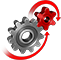# WolframSystemModeler

## DC Motor: Real‐Time Simulation

SystemModeler 4 gives users the possibility to control simulations directly from Mathematica. This example shows how a DC motor reacts to inputs from a user in real time.

To run this example, you'll need

### DC Motor Model

The physical model is a model of a DC motor, combining electrical and mechanical elements. The speed is read by a sensor, and the output is fed back to the user.The motor starts with zero initial speed, and the motor then tries to adjust to the constant speed input, one, as fast as possible. The user input to the system is then added to the reference speed, and the motor will try to adjust to the reference speed.
The model of the motor is easily connected to inputs from Mathematica with the real‐time interface.
A dynamic input variable is provided to the simulation and is visualized in real time, together with the output from the experiment.

Real‐time simulations

Control an experiment in real time with input from Mathematica.

## Wolfram System Modeler

Questions? Comments? Contact a Wolfram expert »